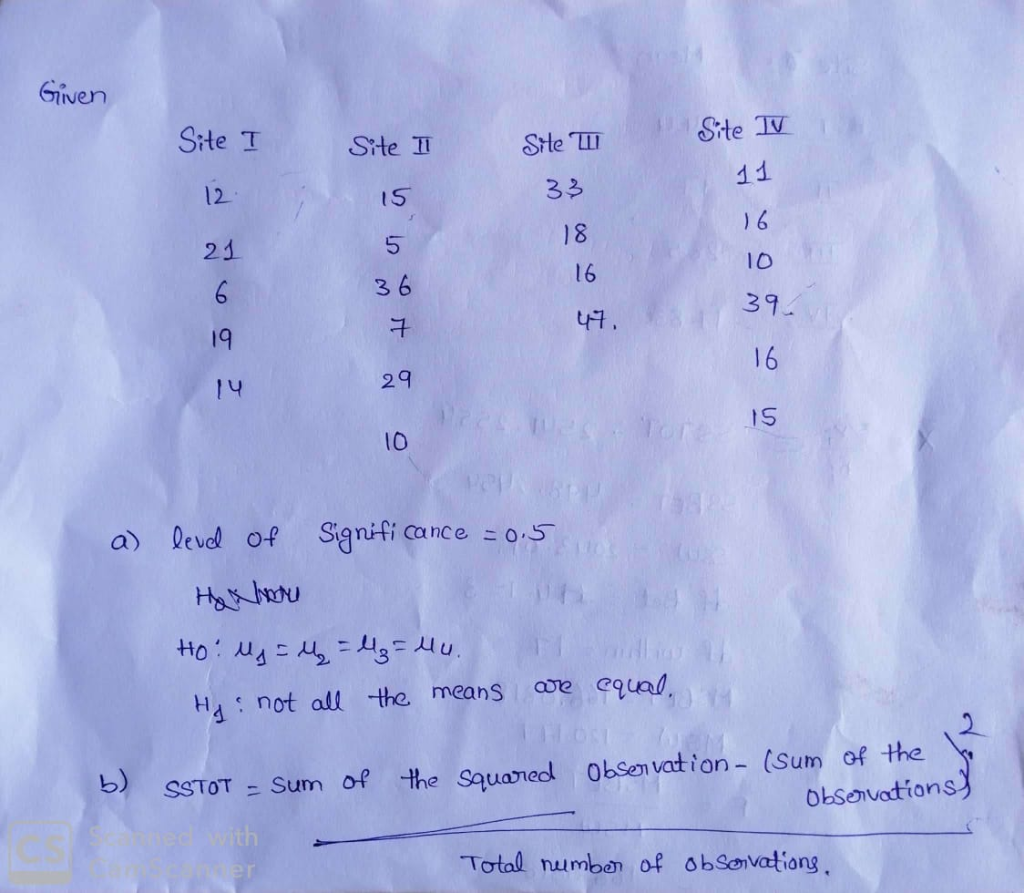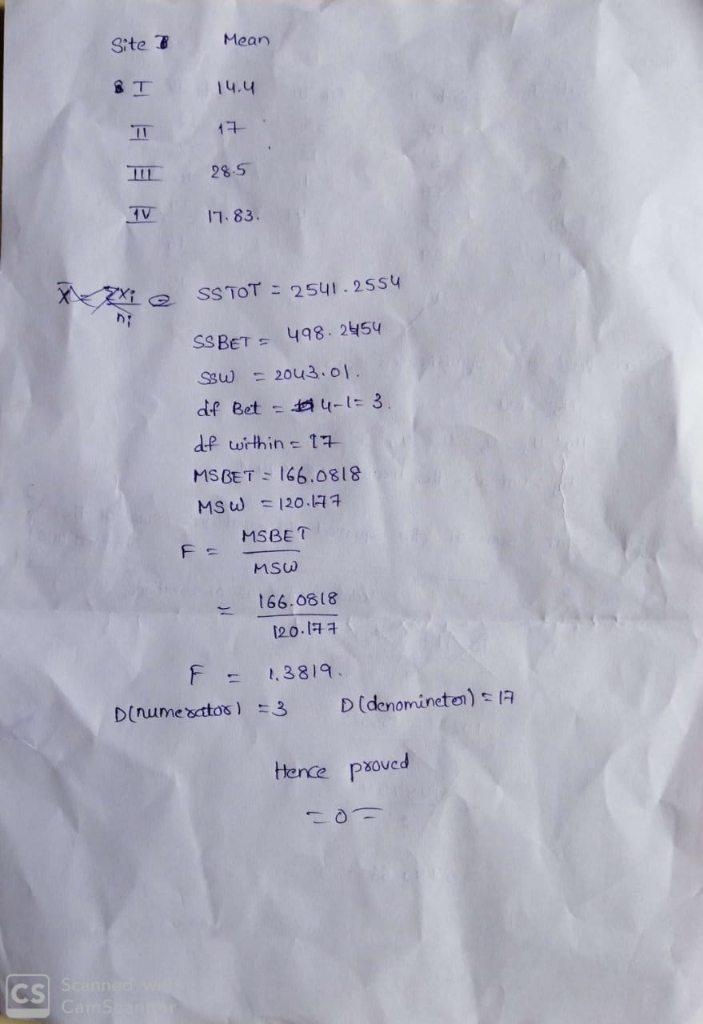Homework Help Question & Answers

# Another type of painted ceramic vessel is called three-circle red-on-white ( Mimbres Mogollon Archaeology). At four different sites in an archaeological region, the number of such sherds was counted i...

Another type of painted ceramic vessel is called three-circle red-on-white ( Mimbres Mogollon Archaeology). At four different sites in an archaeological region, the number of such sherds was counted in local dwelling excavations. Site I Site II Site III Site IV 12 15 33 11 21 5 18 16 6 36 16 10 19 7 47 39 14 29 16 10 13 Shall we reject or not reject the claim that there is no difference in the population mean three-circle red-on-white sherd counts for the four sites? Use a 5% level of significance. (a) What is the level of significance? State the null and alternate hypotheses. Ho: μ1 = μ2 = μ3 = μ4; H1: All four means are different. Ho: μ1 = μ2 = μ3 = μ4; H1: Exactly two means are equal. Ho: μ1 = μ2 = μ3 = μ4; H1: Not all the means are equal. Ho: μ1 = μ2 = μ3 = μ4; H1: Exactly three means are equal. (b) Find SSTOT, SSBET, and SSW and check that SSTOT = SSBET + SSW. (Use 3 decimal places.) SSTOT = SSBET = SSW = Find d.f.BET, d.f.W, MSBET, and MSW. (Use 3 decimal places for MSBET, and MSW.) dfBET = dfW = MSBET = MSW = Find the value of the sample F statistic. (Use 3 decimal places.) What are the degrees of freedom? (numerator) (denominator##### Add Answer of: Another type of painted ceramic vessel is called three-circle red-on-white ( Mimbres Mogollon Archaeology). At four different sites in an archaeological region, the number of such sherds was counted i...
More Homework Help Questions Additional questions in this topic.

• #### Two four-sided dice, one red and one white, will be rolled. List the possible values for the following random variable. Let Y = difference between the number on the red die and the number on the white...

Need Online Homework Help?

Get FREE EXPERT Answers
WITHIN MINUTES
Related Questions